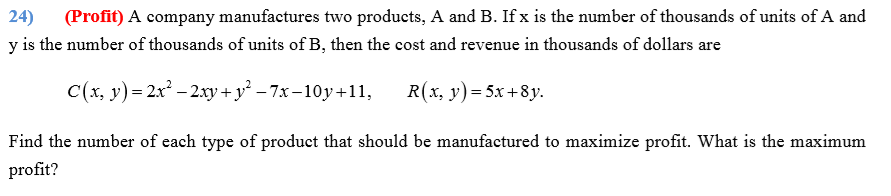# (Profit) A company manufactures two products, A and B. If x is the number of thousands of units of A and 24) y is the number of thousands of units of B, then the cost and revenue in thousands of dollars are C(x, y)= 2x – 2.xy+ y² – 7x-10y+11, R(x, y)= 5x+8y. Find the number of each type of product that should be manufactured to maximize profit. What is the maximum profit?

Question

A company manufactures two products, A and B. If x is the number of thousands of units of A and y is the number of thousands of units of B, then the cost and revenue in thousands of dollars are
C(x,y)=2x2-2xy+y2-7x-10y+11

R(x,y)=5x+8y
Find the number of each type of product that should be manufactured to maximize profit. What is the maximum profit?help_outlineImage Transcriptionclose(Profit) A company manufactures two products, A and B. If x is the number of thousands of units of A and 24) y is the number of thousands of units of B, then the cost and revenue in thousands of dollars are C(x, y)= 2x – 2.xy+ y² – 7x-10y+11, R(x, y)= 5x+8y. Find the number of each type of product that should be manufactured to maximize profit. What is the maximum profit? fullscreen

### Want to see this answer and more?

Experts are waiting 24/7 to provide step-by-step solutions in as fast as 30 minutes!*

*Response times vary by subject and question complexity. Median response time is 34 minutes and may be longer for new subjects.
Tagged in
MathCalculus

### Derivative